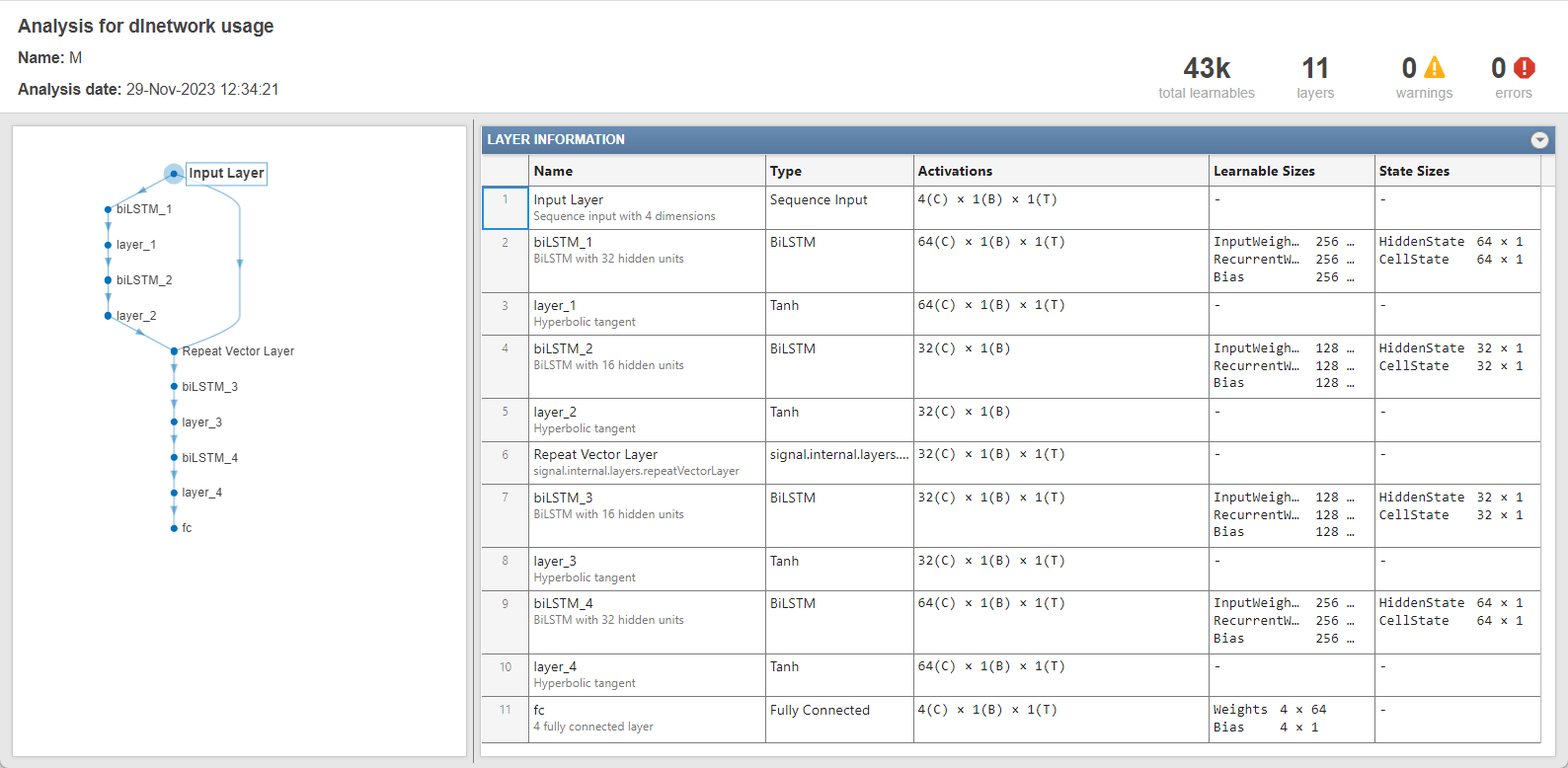# deepSignalAnomalyDetectorLSTM

Detect signal anomalies using LSTM autoencoder

Since R2023a

## Description

The `deepSignalAnomalyDetectorLSTM` object uses a long short-term memory (LSTM) autoencoder model to detect signal anomalies.

## Creation

Create a `deepSignalAnomalyDetectorLSTM` object using `deepSignalAnomalyDetector` and specifying `"lstm"` as the model type.

## Properties

expand all

### General

Training status, specified as `0` (`false`) or `1` (`true`). When the detector has been trained, `IsTrained` is equal to `1`.

Data Types: `logical`

Number of channels in each input signal, specified as a positive integer.

Data Types: `single` | `double` | `int8` | `int16` | `int32` | `int64` | `uint8` | `uint16` | `uint32` | `uint64`

### Model

Type of deep learning model implemented by the detector, specified as `"lstm"`.

Number of hidden units for each layer in the encoder, specified as a vector of positive integers. The ith element of the vector sets the number of hidden units in the ith layer.

Data Types: `single` | `double` | `int8` | `int16` | `int32` | `int64` | `uint8` | `uint16` | `uint32` | `uint64`

Number of hidden units in each layer of the decoder, specified as a vector of positive integers. The ith element of the vector sets the number of hidden units in the ith layer.

Data Types: `single` | `double` | `int8` | `int16` | `int32` | `int64` | `uint8` | `uint16` | `uint32` | `uint64`

### Window

Window length of each signal segment, specified as a positive integer or as `"fullSignal"`.

• If `WindowLength` is specified as an integer, the detector divides each input signal into segments. The length of each segment is equal to the specified value in samples.

• If `WindowLength` is specified as `"fullSignal"`, the detector treats each input signal as a single segment.

Use `updateDetector` to modify this and other window properties.

Data Types: `single` | `double` | `int8` | `int16` | `int32` | `int64` | `uint8` | `uint16` | `uint32` | `uint64` | `char` | `string`

Number of overlapped samples between window segments, specified as a positive integer or as `"auto"`.

• If `OverlapLength` is specified as integer, the detector sets the number of overlapped samples equal to the specified value.

• If `OverlapLength` is specified as `"auto"`, the detector sets the number of overlapped samples equal to `WindowLength` – 1.

Data Types: `single` | `double` | `int8` | `int16` | `int32` | `int64` | `uint8` | `uint16` | `uint32` | `uint64` | `char` | `string`

Method to aggregate sample loss within each window segment, specified as one of these:

• `"max"` — Compute the aggregated window loss as the maximum value of all the sample losses within the window.

• `"mean"` — Compute the aggregated window loss as the mean value of all the sample losses within the window.

• `"median"` — Compute the aggregated window loss as the median value of all the sample losses within the window.

• `"min"` — Compute the aggregated window loss as the minimum value of all the sample losses within the window.

Data Types: `char` | `string`

### Threshold

Method to compute the detection threshold, specified as one of these:

• `"contaminationFraction"` — Value corresponding to the detection of anomalies within a specified fraction of windows. The fraction value is specified by `ThresholdParameter`.

• `"max"` — Maximum window loss measured over the entire training data set and multiplied by `ThresholdParameter`.

• `"median"` — Median window loss measured over the entire training data set and multiplied by `ThresholdParameter`.

• `"mean"` — Mean window loss measured over the entire training data set and multiplied by `ThresholdParameter`.

• `"manual"` — Manual detection threshold value based on `Threshold`.

• `"customFunction"` — Custom detection threshold value based on `ThresholdFunction`.

Use `updateDetector` to modify this and other threshold properties.

Data Types: `char` | `string`

Detection threshold, specified as a nonnegative scalar when `ThresholdMethod` is set to `"contaminationFraction"`, and as positive scalar when `ThresholdMethod` is set to `"max"`, `"median"`, or `"mean"`.

Data Types: `single` | `double` | `int8` | `int16` | `int32` | `int64` | `uint8` | `uint16` | `uint32` | `uint64`

Manual detection threshold, specified as a positive scalar. This property applies only when `ThresholdMethod` is set to `"manual"`.

Data Types: `single` | `double` | `int8` | `int16` | `int32` | `int64` | `uint8` | `uint16` | `uint32` | `uint64`

Function to compute custom detection threshold, specified as a function handle. This property applies only when `ThresholdMethod` is set to `"customFunction"`.

Data Types: `function_handle`

## Object Functions

 `detect` Detect anomalies in signals `getModel` Get underlying neural network model of signal anomaly detector `plotAnomalies` Plot signal anomalies `plotLoss` Plot window reconstruction loss `plotLossDistribution` Plot CDF and histogram of aggregated window loss distribution `trainDetector` Train signal anomaly detector `updateDetector` Update settings of trained detector and recompute detection threshold

## Examples

collapse all

Create an object that uses a long short-term memory (LSTM) autoencoder model to detect anomalies in four-channel signals. Use `deepSignalAnomalyDetector` with default property values.

`D = deepSignalAnomalyDetector(4,"lstm");`

Get the underlying neural network model of the LSTM anomaly detector. Use the `analyzeNetwork` (Deep Learning Toolbox) function to inspect the model.

```M = getModel(D); analyzeNetwork(M)```## Version History

Introduced in R2023a